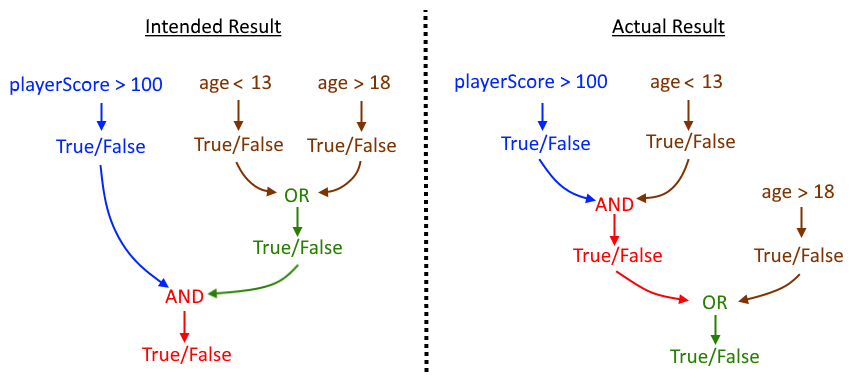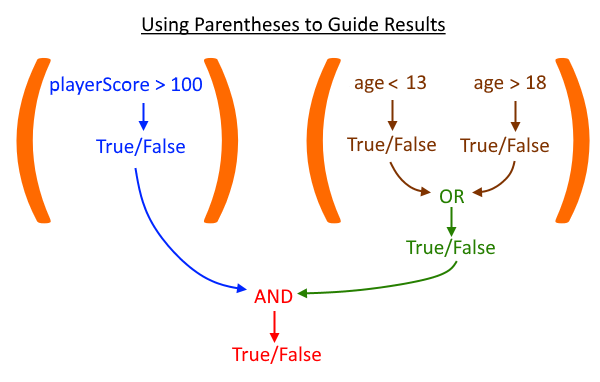# Chapter Four: Making Decisions

## Lesson Four: More Complex Expressions

Many of your logical expressions will simply contain a few, clear phrases. For example, the expression "A < B or C >= D" clearly says to "or" together the results of the conditions "A < B" and "C >= D". However, you may need to chain together multiple operators to test for increasingly complicated conditions. When that happens, if Python doesn't execute the individual operators in the order you desire, the results can be unexpected.

### Uncertain Evaluation Order

Imagine that you want to run a block of code when both of the following conditions are True:

• playerScore > 100
• age < 13 or age > 18

So, the overall expression should be True if the playerScore is greater than 100 and the age is less than 13 orgreater than 18. You might begin to write a Python "if" statement as follows:

if (playerScore > 100 and age < 13 or age > 18):

How will Python interpret this code? Will it test "playerScore > 100" first, then "and" together the results of "age > 13" or "age <= 18" next, and finally "or" together the results as you intended? That evaluation path is shown on the left side of the image below.Or, will the code actually test "playerScore > 100" first, then "and" that result to "age < 13", and finally "or" that result to "age > 18"? That evaluation order can produce an entirely different result, as shown on the right side of the image above. Python does not know what you intended, only what you have written in the code.

Without any more guidance from you, the programmer, Python will follow its own internal rules for evaluating expressions in a default order. There's a great chance that default order doesn't meet your program's needs, so you will want to use parentheses to make it clear to Python - and anyone reading your code - exactly how the expression will be evaluated.

### Using Parentheses with Logical Operators

Any time you surround an operation in parentheses ( ), that tells Python to evaluate the operation first. Then, take the result and use it in any surrounding expression outside the parentheses. Let's re-write our example with parentheses to make sure it works correctly.

if ((playerScore > 100) and (age < 13 or age > 18)):

With these parentheses, we have told Python to evaluate "playerScore > 100" and "age < 13 or age > 18" first, independently. Those True or False results are then combined with "and" to produce the final answer.It is best practice to always use parentheses to make your logic perfectly clear! That way, both Python and anyone else that reads your code will understand your intent and evaluate the expression correctly.

Experiment with the sample code below. Try setting different values for the playerScore and age variables, then see the results from logical expressions that are written with and without the guiding parentheses.

Try It Now

Try changing the initial values for playerScore and age to make each of the individual comparisons True or False. Can you find a combination where the two results are not the same? If the playerScore is below 100 and the ageis above 18, for example, these two expressions give different answers. Only the second expression, when parentheses are used, will always produce results to match our original logic requirements.

### Review - Mathematical, Comparison and Logical Operators

You have now seen quite a few Python symbols that let you perform mathematical operations, compare values, or join Boolean expressions. It's easy to get them confused, so let's quickly review each group of operators.

Mathematical Operators:

Mathematical operators are used to add, subtract, multiply and divide numeric values to produce a numericresult.

SymbolDescriptionExample Statement
-SubtractionnumericResult = 5 - 3
*MultiplicationnumericResult = 4 * 2
/DivisionnumericResult = 10 / 5

Comparison Operators:

Comparison operators are used to compare two values of any type and produce a Boolean (True/Falseresult.

SymbolDescriptionExample Statement
==Equal TobooleanResult = 1 == 2
!=Not Equal TobooleanResult = 1 == 2
<Less ThanbooleanResult = 1 < 2
<=Less Than or Equal TobooleanResult = 1 <= 2
>Greater ThanbooleanResult = 1 > 2
>=Greater Than or Equal TobooleanResult = 1 >= 2

Logical Operators:

Logical operators are used to join two Boolean True/False values or flip a single Boolean value.

SymbolDescriptionExample Statement
andTrue if A AND B are TruebooleanResult = (1 == 2) and (3 < 4)
orTrue if A OR B are TruebooleanResult = (1 == 2) or (3 < 4)
notTrue if A is False or False if A is TruebooleanResult = not (1 > 2)

### Mixing Operators and Parentheses

You can write very lengthy logical expressions with careful use of parentheses. Just make sure that you are applying mathematical operators to numeric values, comparison operators to any type of data, and logical operators to Boolean variables!

You can also use parentheses to help clarify mathematical expressions. What will happen when you write the following expression?

result = 1 + 3 * 5

Will "1 + 3" happen first giving you 4, then that result is multiplied by 5 to reach 20? Or, will 3 * 5 happen first giving you 15, then 1 is added to that result to reach 16?

Just as with logical expressions, Python has a default evaluation order for mathematical operators, and it is not necessarily left-to-right. So, instead of trying to understand and apply the internal Python rules, it is best practice to always use parentheses with your mathematical operations to make sure they behave exactly the way you want. The example below uses parentheses to force the "1 + 3" operation to happen first, and therefore the resulting value is 20.

result = (1 + 3) * 5

Now, let's explore a few more examples that use a mixture of mathematical, comparison and logical operators. Each of the expressions below is valid and will produce a Boolean result. Can you figure out what the result will be in each case, before you run the example code?

Try It Now

You are encouraged to experiment with the initial values and the expressions themselves to form new results. Can you correctly predict the result each time you make a change?

When using parentheses, every opening parenthesis "(" must be matched by exactly one closing parenthesis ")" somewhere in that same statement. Carefully ensure you have the same number of opening and closing parentheses in every statement, and that each matched pair clearly surrounds the expression or expressions that you want to mark.

### Reminder - Breaking Long Statements across Multiple Lines

When writing long logical expressions, you might not be able to fit the entire expression on one code line comfortably. Remember that Python allows you to break a long statement across multiple lines. Simply add a backslash (\) to mark a split and continuation of that statement on the next line.

The following two code examples each contain a single statement that does the same thing.

result = (A < 3) or (B > 5) and ((C + D) == 42)
result = (A < 3) or \
(B > 5) and \
((C + D) == 42)

You may find one version easier to read than the other. You can decide to break long lines at certain spots (or not) in ways that make sense to you.

Work with Me: Prize Winner

Your math club has set up a raffle, where raffle ticket is a winner if it has a series of numbers that meet some specific mathematical requirements. In this exercise, you are going to write the "if" statement and logical expressions that will determine if a series of numbers from a ticket is a winning number and print out a message to the screen.

As you gain experience with Python, you will be expected to write more code yourself based on activity requirements, instead of simply filling small blank spots in code that we give you. For this exercise, we'll describe the program requirements and start you off with just enough code to define the ticket numbers. The rest of the code ("if" statement, logical expressions, print() statements, etc.) will be up to you!

Program Requirements

The program will start with 6 ticket numbers held in variables number1 through number6; each number should be a single digit from 0 through 9. A ticket is declared the winner if ALL the following conditions are True:

• number1 is greater than number6
• number2 multiplied by number3 is greater than 20
• number4 minus (number5 * number1) is less than 0

If all the above conditions are True, the program should print "Winner!", else it should print "Thanks for your contribution to the math club".

Now that you understand the program requirements, go ahead and complete the code below.

Try It Now

Console

The default ticket numbers used above - 3, 5, 2, 4, 8, 0 - should not produce a winning ticket. However, the numbers 8, 6, 7, 5, 3, 0 should produce a winner! There may be many other number combinations that are also winning tickets.

Try entering a variety of numeric combinations to see how often you can make winning raffle ticket numbers. Based on your experience, do you think the math club was too generous when setting up the winning formula? What changes might you make to the raffle requirements to produce a smaller set of winners?

End of Lesson